# Civil Engineering - Hydraulics

### Exercise :: Hydraulics - Section 1

21.

A cylinder 3 m in diameter and 4 m long retains water one side as shown in the below figure. If the weight of the cylinder is 2000 kgf, the horizontal reaction at B is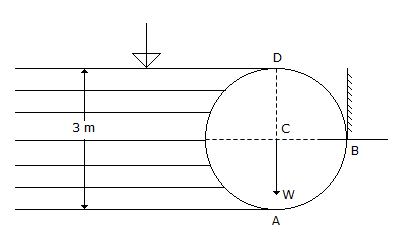A. 10,000 kgf B. 15,000 kgf C. 20,000 kgf D. 18,000 kgf.

Explanation:

No answer description available for this question. Let us discuss.

22.

Inside pressure in a hollow soap bubble in the air is : (where d is the diameter of the bubble)

 A.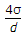B.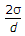C.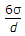D.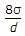Explanation:

No answer description available for this question. Let us discuss.

23.

The momentum correction factor (β) for the viscous flow through a circular pipe is

 A. 1.25 B. 1.33 C. 1.5 D. 1.66 E. 2

Explanation:

No answer description available for this question. Let us discuss.

24.

A piezometer opening in pipes measures

 A. velocity head B. static pressure C. total pressure D. negative static pressure.

Explanation:

No answer description available for this question. Let us discuss.

25.

An independent mass of a fluid does not posses

 A. elevation energy B. kinetic energy C. pressure energy D. none of these.